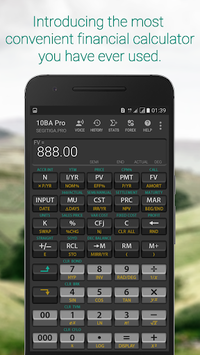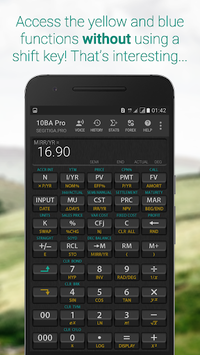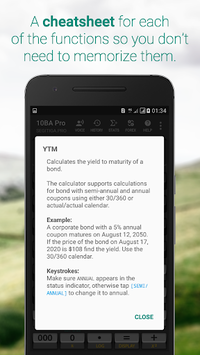# 10BA Professional Financial Calculator APK

Like the industry standard financial calculator, but with TONS of extra features

### APK Details

 Category Productivity Last Updated 10-26-2018 Latest Version 1.1g Size 9.6 MB Installations 50,000+ downloads Android version 4.1 and up Content Rating Everyone Ad Supported false Date Released Oct 8, 2017

### Screenshots## 10BA Professional Financial Calculator APK Description

The 10BA Financial Calculator works like the industry standard financial calculator such as the HP 10B, HP 10BII, or the HP 10BII+ emulator; however, we add tons of unique features that makes this app be the most convenient financial calculator that you have ever used.

Single-Access Key
Now you do not need to use a shift key to access the yellow or the blue functions. The Single-Access Key feature enables you to activate the yellow and the blue functions quickly and conveniently.

Integrated Cheatsheet
You don't have to memorize anything. Each of the functions on the calculator has a cheatsheet containing brief description of how the function works, and one or more examples.

Anti-Boring
Are you bored with the look of current calculator? With the 10BA Pro Financial Calculator you can replace the background with an image from your photo gallery. You can even make it transparent like the interface you often see on TV, kind of.

Zero-Learning Curve
If you are already familiar with the 10BII+ or similar financial calculator, practically there is no learning curve with the 10BA Pro. We add features that makes it even easier to use than the real thing.

Peace of Mind
The 10BA Pro Financial Calculator has hundreds of functions and many of them you'll probably never use. But you know they are there, in case you need them.

Integrated Currency Converter
Currency converter is integrated into the 10BA Pro Financial Calculator and is only a keystroke away.

Statistical Distribution Functions
The 10BA Pro Financial Calculator has a separate screen for the statistical functions so many statistical functions can be accomodated. It can calculate most distribution functions that you find at the back of a statistics book.

Amortization Schedule
Produce an amortization schedule that you can convert into Excel format and later share with your contacts.

Financial Functions
• TVM: Number of periods, Interest/year, Present value, Periodic payment, Future value, Amortization, Amortization schedule
• Business percentages: Percent, Percent change, Markup, Cost, Price, Margin
• Cashflow analysis: IRR, MIRR, Net present value, Net future value
• Depreciation: Straight line, Sum of the years’ digits, Declining balance
• Interest conversion: Nominal, Effective
• Break even analysis: Units, Sales price, Variable cost, Fixed cost, Profit
• Bonds: Accrued interest, YTM, Price, Coupon, Call, 360/Actual, Semiannual/Annual
• Calendar functions: Date, Date difference, 360/Actual

Statistical Functions
• Summary statistics
• Regressions: Linear, Exponential, Logarithmic, Power, Exponent, Inverse
• X-Estimates, Y-Estimates, Correlation
• Standard deviation: Sample, Population
• Average, Weighted average
• Permutation, Combination
• Distribution: Binomial, Poisson, Normal, Inverse Normal, Student-t, Inverse Student-t, Chi-Square, Inverse Chi-Square, F, Inverse F
• Pseudo random number

Mathematical functions
• Basic arithmetic
• Trigonometric, Inverse trigonometric
• Hyperbolic, Inverse Hyperbolic

Feedback and Contact Info